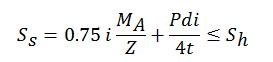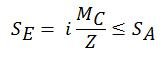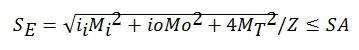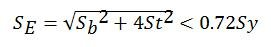# Flexibility Stresses: Code Equations

Flexibility stresses

The piping system experiences different type of stresses when it is installed, tested and in operation. There are different codes according to which the system is designed, each code has different formula for calculating the stress induced in the piping system. Below mentioned are the code equations:

## 1. ANSI B 31.1 – Power Piping

The Power Piping Code ANSI B 31.1 specifies that the developed stresses due to sustained, occasional and expansion stresses be calculated in the following manner.

• 1.1 SustainedWhere, Ss = Sustained stress

i     = Stress Intensification factor

MA= Resultant moment due to Primary loadsSh = Basic allowable stress at the operating temperature

Z   = Stress Intensification factor

• 1.2 OccasionalWhere, So  = Occasional stress

i     = Stress Intensification factor

MB = Resultant moment due to occasional loadsK   = Stress Intensification factor

= 1.2 for loads occurring less than 1% of the time

= 1.15 for loads occurring less than 10% of the time

• 1.3 ExpansionWhere, SE  = Expansion stress range

MC = Resultant moment due to expansion (Secondary) loadsSA  = Allowable e+xpansion stress range

## 2. ANSI B 31.3 – Process Piping

• 2.1 Sustainediiio = In-plane & out-plane stress intensification factor

Sh= Basic allowable stress at the operating temperature

• 2.2 Occasional

The code states that calculate the stresses due to sustained and occasional loads independently as per the above equation and then add them absolutely. The sum should not exceed 1.33 Sh.

• 2.3 ExpansionWhere, Mi  =Range of inplane bending moment due to expansion (secondary) load

Mo  = Range of outplane bending moment due to expansion (secondary)load

MT  = Range of torsional bending moment due to expansion load

SE = Expansion stress range

SA   = Allowable stress range

## 3. ANSI B 31.4 – Fuel gas piping

The code requires that the sustained, operating, expansion & occasional stresses be calculated and checked as below.

• 3.1 SustainedWhere, Slp = Longitudinal pressure stressSb  = Bending stress due to sustained loads

• 3.2 ExpansionWhere, Sb  = Range of bending stress due to varying loadsSt = Range of torsional stress due to varying loadsSy = Specified Minimum Yield stress

• 3.3 OperatingWhere, F = % of axial restraint

= 1 for buried pipe lines

= 0 for above ground pipelines

E = Modulus of Elasticity

a = Thermal expansion coefficient of pipe material

dT= Temperature change from ambient

V = Poisson’s Ratio

SH = Hoop stress =• 3.4 OccasionalWhere, Slp = Longitudinal pressure stress

Sb  = Resultant moment due to occasional loads

## 4. ANSI B 31.4 – Fuel gas piping

Like B 31.4 code B 31.8 also requires calculations and check of sustained, expansion, operating and occasional stress.

• 4.1 SustainedWhere, Slp = Longitudinal pressure stressSb  = Bending stress due to sustained loads

Sy = Specified Minimum Yield of pipe material

F  = Factor for construction type specified in table 841.114A

T  = Temperature derating factor specified in 841.116A

• 4.2 ExpansionWhere, Sb  = Bending stress due to varying loads

St  = Range of torsional stress due to varying loads

• 4.3 Operating• 4.4 OccasionalWhere, K  = Occasional load factor